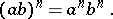# Power

(diff) ← Older revision | Latest revision (diff) | Newer revision → (diff)

In its primary meaning (an integer and positive power), it is the product of a number of equal factors and is written in the form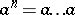(times), whereis the base,the exponent and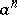the power. The basic properties of powers are:Further generalizations of the idea of a power include: zero powers: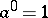(when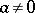); negative powers: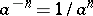; fractional powers: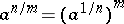; and a power with an irrational exponent: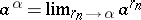, where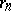is an arbitrary sequence of rational numbers tending to. Powers with a complex base (see de Moivre formula) and powers with a complex base and complex exponent (by definition: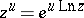) are also studied.